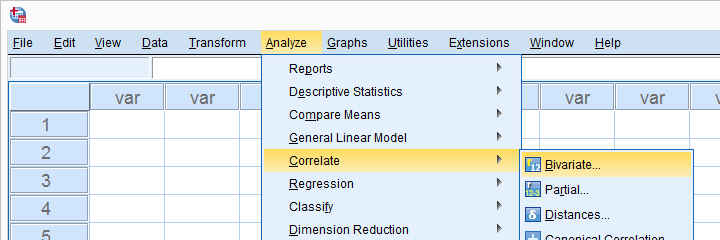SPSS TUTORIALS BASICS ANOVA REGRESSION FACTOR CORRELATION

# Overview SPSS Correlation Tutorials## Pearson Correlations

### Creating APA Style Correlation Tables in SPSS

How to create an APA format correlation matrix in SPSS? This simple tutorial shows the easy way to do so and offers a Python script that processes one or many tables in one go.

### SPSS Correlation Analysis Tutorial

This tutorial quickly walks you through the correct steps for a correlation analysis in SPSS.

We'll cover a quick data check, the assumptions, significance levels, APA reporting and more.

### Pearson Correlations – Quick Introduction

A Pearson correlation is a number between -1 and +1 that indicates how strongly two variables are linearly related.

This simple tutorial quickly explains the basics with outstanding illustrations and examples.

## Kendall's Tau

### Kendall’s Tau – Simple Introduction

Kendall’s Tau is a number between -1 and +1 that indicates to what extent 2 variables are monotonously related.

This tutorial quickly walks you through some basics such as assumptions, significance and confidence intervals for Kendall’s Tau.

### Kendall’s Tau in SPSS – 2 Easy Options

Kendall’s Tau-B is found in SPSS under AnalyzeCorrelateBivariate

Alternatively, both Kendall’s Tau-B and Tau-C can be obtained from AnalyzeDescriptive statisticsCrosstabs

Be aware that this second option may report incorrect significance levels for small sample sizes.

## Association Measures

### Cramér’s V – What and Why?

Cramér’s V is a number between 0 and 1 that indicates how strongly two nominal variables are correlated.

Because it's suitable for categorical variables, Cramér’s V is often used as an effect size measure for a chi-square independence test.

### SPSS – Kendall’s Concordance Coefficient W

Kendall’s Concordance Coefficient W is a number between 0 and 1 that indicates interrater agreement.

This tutorial explains the basic idea behind Kendall’s W and shows how to get it from SPSS.

## Spearman Rank Correlations

### Spearman Rank Correlations – Simple Tutorial

A Spearman rank correlation is a number between -1 and +1 that indicates to what extent 2 variables are monotonously related.

This tutorial quickly walks you through the basics such as assumptions, significance levels, software and more.

## Correlation Tutorials - Other

### SPSS CORRELATIONS – Beginners Tutorial

SPSS CORRELATIONS creates tables with Pearson correlations, sample sizes and significance levels.

Its syntax can be as simple as correlations q1 to q5. which creates a correlation matrix for variables q1 through q5. This simple tutorial quickly walks you through some other options as well.

# Tell us what you think!

*Required field. Your comment will show up after approval from a moderator.

# THIS TUTORIAL HAS 4 COMMENTS:

•### By Vaishali on October 4th, 2018

Hi

Thank you for such an easy and amazing way of reporting and making tables in SPSS in line with APA style.
If you can please can you share a similar kind of document for Partial correlation?

Waiting to hear soon.

-Vaishali

•### By Ruben Geert van den Berg on October 4th, 2018

Hi Vaishali!

Thanks for the compliment. We haven't covered (semi)partial correlations yet. We aren't planning to do so any time soon either. It's a rather specialist topic for a small audience. We first like to expand our SPSS Beginners Tutorials.

Hope that helps!

SPSS tutorials

•### By aya on September 2nd, 2020

thank you for the amazing and clear illustration. I have one question however, what test of correlation should I use to study association between one nominal variable and one continuous? For example, association between grade and occupation, marital status, smoking status... ?
thank you

•### By Ruben Geert van den Berg on September 3rd, 2020

Great question!

You'd normally test if the mean scores of the quantitative variable are equal over all levels of the categorical variable with an ANOVA.

Now, the effect size for ANOVA is (partial) eta squared. This is the proportion of variance accounted for and thus has the same meaning as a (squared) Pearson correlation.

You'll also find this in the table presented in Which Statistical Test Should I Use? under "quantitative" and "nominal".

Hope that helps!

SPSS tutorials